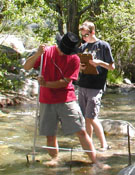# Calculation of Stream Discharge Required to Move Bed MaterialReed Schwimmer
,
Rider University
Author Profile

#### Summary

Students will measure stream velocity, depth, and grain sizes across two channel transects. After constructing both a longitudinal profile and two cross sections, students will calculate the discharge required to move the bed load.

Used this activity? Share your experiences and modifications

## Context

#### Audience

This activity is used in the Sedimentology course, a 300-level geology course.

#### Skills and concepts that students must have mastered

Students need to be able to construct cross sections, measure flow velocities, and estimate grain sizes.

#### How the activity is situated in the course

The activity is a stand-alone exercise, but its strength is the field application (i.e., collecting, analyzing, and interpreting data) of previously covered information to answer a practical geologic problem.

## Goals

#### Content/concepts goals for this activity

The goal is to show the students how geomorphic features relate to physical processes and how these processes can be examined quantitatively.

#### Higher order thinking skills goals for this activity

The thinking skills involve setting up the field work so the necessary data can be collected. Students will need to quantitatively analyze the data to relate theoretical relationships to geomorphic processes and features.

#### Other skills goals for this activity

Other skills involve using an auto level and a digital current meter.

## Description of the activity/assignment

Students will map two sections of a stream and calculate the depth, velocity, and discharge of flows required to move the stream bedload. Students will produce two cross-sectional profiles of the stream, one through a pool and one through a riffle section, and one longitudinal profile. Measurements of sediment size, depth, and velocity, will be determined at meter intervals across each cross transect. Students will also calculate the approximate cross-sectional areas across the pool and riffle sections and associated discharges. Students will also determine the stream gradient along the longitudinal profile. From these data, students will employ hydrodynamic equations to calculate the critical shear stress, and mean flow velocity required to move the bedload. Students can then calculate what the discharge and stream width would be at the time of sediment movement, and compare these data with those calculated for "normal-flow" periods.

## Determining whether students have met the goals

I grade the maps and profiles the students produce, their quantitative results, and their conceptual answers to associated questions.